Worksheet Questions: Algebra

# Worksheet Questions: Algebra - Notes | Study Mathematics (Maths) Class 6 - Class 6

 1 Crore+ students have signed up on EduRev. Have you?

Q.1. Find the rule which gives the number of lines required to make the following pattern. Use a variable to write the rule.

(i) Letter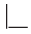__________

(ii) Letter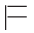__________

(iii) Letter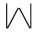__________

(iv) Letter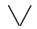__________

Ans.
(i) 2n
(ii) 3n
(iii) 4n
(iv) 2n

Q.2. Children stand 10 in a row to perform a drill. How many children can there be in a drill? Give the rule to calculate this. (Use n for the number of rows)

Ans. 10 n

Q.3. Form an algebraic expression using the statements given below :
(i) 17 subtracted from '–y' __________
(ii) '–m' multiplied by 10 __________
(iii) x divided by '–2' __________
(iv) 4 added to 6 times y __________

Ans.
(i) – y – 17
(ii) – 10m

(iii)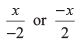(iv) 6y + 4

Q.4. If y is Meena's age now in years how old will she be after 7 years? __________

Ans. (y + 7) years

Q.5. If the side of a regular septagon is m units, then what is it's perimeter? __________

Ans. 7 m units

Q.6. Is 2 p–3> 7 an algebraic equation? __________

Ans. No

Q.7. A teacher distributes 6 notebooks per student. How many notebooks are needed if s is the number of students?

Ans. 6s

Q.8. Pick out the correct solution from the values given in the bracket next to each equation:

(i) r – 8 = 0 (8, – 8, 0, 1) r = __________

(ii)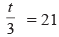(7, 14, 21, 63) t =          __________

(iii)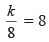(1, –1, 64) k =        __________

Ans. (i) r = 8
(ii) t = 63
(iii) k = 64

Q.9. Complete the table and by inspection of the table find the solution to the equation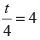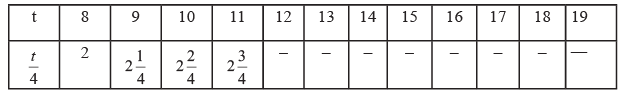Ans.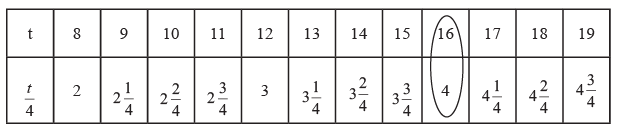t = 16

Q.10. In a game of cricket, there are n children but in football there are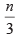children. What does this indicate?

Ans. It indicates that the number of children in the game of football is one-third as there are in the game of cricket.

Q.11. Match the following :
(i) 5x = 20 (a) x = 6

(ii) 8 = x + 3 (b) x = 4

(iii)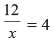(c) x = 5

(iv) 8 – x = 2 (d) x = 3

Ans.
(i) ↔ (b)
(ii) ↔ (c)
(iii) ↔ (d)
(iv) ↔ (a)

Q.12. Give a mathematical expression for the statement: m multiplied by 5 and 6 subtracted from the product.

Ans. 5 m – 6

Q.13.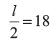l = __________

Ans. l = 36

Q.14. n – 12 = – 19  n = __________

Ans. n = – 7

Q.15.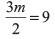m = __________

Ans. m = 6

The document Worksheet Questions: Algebra - Notes | Study Mathematics (Maths) Class 6 - Class 6 is a part of the Class 6 Course Mathematics (Maths) Class 6.
All you need of Class 6 at this link: Class 6

## Mathematics (Maths) Class 6

168 videos|282 docs|43 tests
 Use Code STAYHOME200 and get INR 200 additional OFF

## Mathematics (Maths) Class 6

168 videos|282 docs|43 tests

Track your progress, build streaks, highlight & save important lessons and more!

,

,

,

,

,

,

,

,

,

,

,

,

,

,

,

,

,

,

,

,

,

;